# Accounting Ratios (Part - 2) Notes | Study TS Grewal Solutions - Class 12 Accountancy - Commerce

## Commerce: Accounting Ratios (Part - 2) Notes | Study TS Grewal Solutions - Class 12 Accountancy - Commerce

The document Accounting Ratios (Part - 2) Notes | Study TS Grewal Solutions - Class 12 Accountancy - Commerce is a part of the Commerce Course TS Grewal Solutions - Class 12 Accountancy.
All you need of Commerce at this link: Commerce

Page No. 4.93

Question:26
Quick Ratio of a company is 2:1. State giving reasons, which of the following transactions would

(i) improve,
(ii) reduce,
(iii) Not change the Quick Ratio:
(a)
Purchase of goods for cash;

(b)
Purchase of goods on credit;

(c)
Sale of goods costing 10, 000
for 10,000;

(d)
Sale of goods costing 10, 000
for 11,000;

Solution:

Quick Ratio = 2:1
Let Quick Assets be = Rs 20,000
Current Liabilities = Rs 10,000
(a)
Purchase of goods for Cash- Reduce
Reason: This transaction will result decrease in cash and increases in stock. Liquid Asset will decrease due payment for goods purchased.
Example: Purchase of goods Rs 5,000 for cash
Quick Assets = 20,000 − 5,000 Cash
= Rs 15,000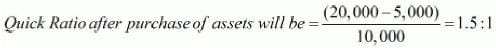(b)
Purchase of goods on Credit- Reduce
Reason: Purchase of goods on credit will result increase in Current Liabilities and no change in Quick Assets.
Example: Purchase of goods on Credit Rs 5,000
Current Liabilities = 10,000 + 5,000 Creditors
= Rs15,000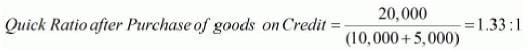(c)
Sale of goods for Rs 10,000- Improve
Reason: Sale of goods will result in increase in Quick Assets by the amount of Rs 10,000 in the form of either in cash or debtor. This transaction will result no change in current liabilities.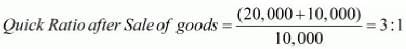(d)
Sale of goods costing Rs 10,000 of or Rs 11,000- Improve
Reason: This transaction will increase the Quick Assets by Rs 11,000 in the form of either in cash or debtors but no effect on the Current Liabilities.
Quick Assets after sale of goods = 20,000 + 11,000 = Rs 31,000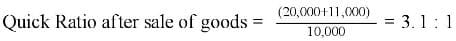(e)
Cash received from debtors- No change
Reason: This transaction results increase in one quick asset in the form of cash and decrease in other quick asset in the form of debtor with equal amount. Therefore it result in no change in the total of Quick Assets.
Example: Cash received from debtors Rs 5,000
Quick Assets = 20,000 + 5,000 (Cash) − 5,000 (Debtors) = 20,000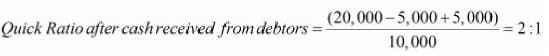Question:27
The Quick Ratio of a company is 0.8:1. State with reason, whether the following transactions will increase, decrease or not change the Quick Ratio: (i) Purchase of loose tools for 2,000; (ii) Insurance premium paid in advance 500; (iii) Sale of goods on credit 3,000; (iv) Honoured a bills payable of 5,000 on maturity.

Solution: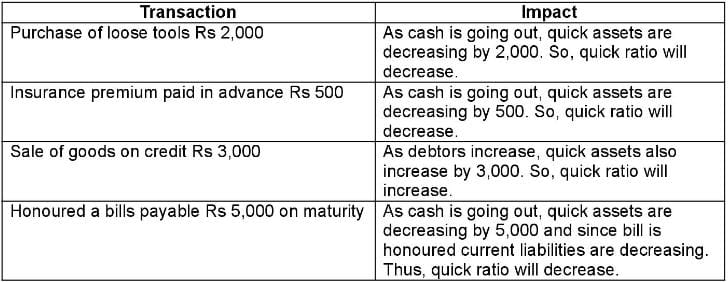Page No. 4.94

Question:28
XYZ Limited's Inventory is 3,00,000. Total Liquid Assts are 12,00,000 and Quick Ratio is 2:1. Work out Current Ratio.
Solution: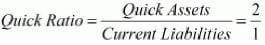Quick Assets = 12,00,000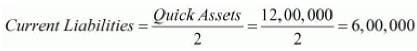Current Assets = Quick Assets + Stock= 12,00,000 + 3,00,000 = 15,00,000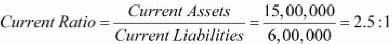Question:29
Total Assets 22,00,000; Fixed Assets 10,00,000; Capital Employed 20,00,000. There were no Long-term Investments. Calculate Current Ratio.
Solution:
Current Assets = Total Assets − Fixed Assets
Fixed Assets = 10,00,000
Total Assets = 22,00,000
∴ Current Assets = 22,00,000 − 10,00,000 = 12,00,000
Current Liabilities = Total Assets − Capital Employed
= 22,00,000 − 20,00,000 = 2,00,000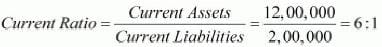Question:30
Capital Employed 10,00,000; Fixed Assets 7,00,000; Current Liablities 1,00,000. There are no Long-term Investments. Calculate Current Ratio.
Solution:

Capital Employed = 10,00,000
Fixed Assets = 7,00,000
Current Assets = Capital Employed + Current Liabilities − Fixed Assets
= 10,00,000 + 1,00,000 − 7,00,000 = 4,00,000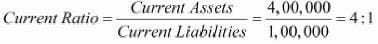Question:31
Following is the Balance Sheet of Crescent Chemical Works Limited as at 31st March, 2019: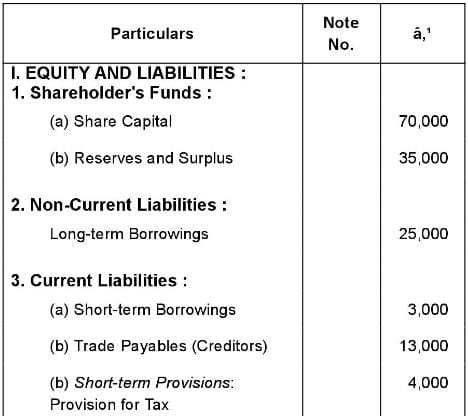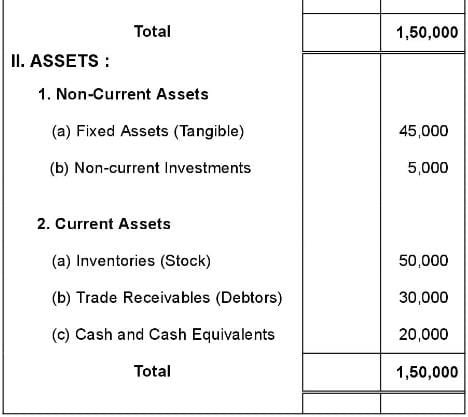Compute Current Ratio and Liquid Ratio
Solution:
Current Assets = Inventory + Trade Receivables + Cash and Cash Equivalents
= 50,000 + 30,000 + 20,000 = 1,00,000
Current Liabilities = Short-term Borrowings + Trade Payables + Provision for Tax
= 3,000 + 13,000 + 4,000 = 20,000
Quick Assets = Trade Receivables + Cash and Cash Equivalents
= 30,000 + 20,000 = 50,000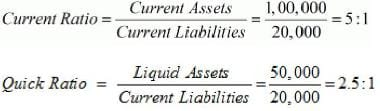1. Ideal Current Ratio for a business is considered to be 2:1. But in this case the ratio is quite high i.e. 5:1. This may be due to the following reasons:
(i) Blockage of Funds in Stock
(ii) High Amount outstanding from Debtors
(iii) Huge Cash and Bank Balances
2. Ideal Quick Ratio of a business is supposed to be 1:1. This implies that Liquid Assets should be equal to the Current Liabilities. But in the given case Quick Ratio is 2.5 : 1 which indicates that the Liquid Assets are quite high in comparison to the Current Liabilities.

Question:32
From the following calculate:
(i) Current Ratio; and
(ii) Quick Ratio: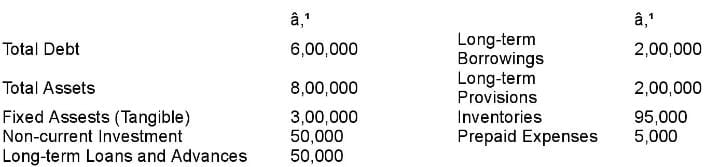Solution:
(i) Current Ratio
Current Assets = Total Assets - Fixed Assets - Non-Current Investment- Long term Loans and Advances
= 8,00,000 - 3,00,000 - 50,000 - 50,000 = Rs 4,00,000
Current Liabilities = Total Debt - Non - Current Liabilities
= 6,00,000 - 2,00,000 - 2,00,000 = Rs 2,00,000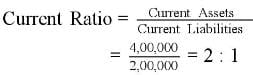(ii) Quick Ratio
Quick Assets = Current Assets - Stock - Prepaid Expenses
= 4,00,000 - 95, 000 − 5, 000 = Rs 3,00,000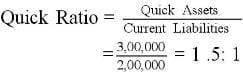Question:33
Calculate Debt to Equity Ratio: Equity Share Capital 5,00,000; General Reserve 90,000; Accumulated Profits 50,000; 10% Debentures 1,30,000; Current Liabilities 1,00,000.
Solution:

Equity = Equity Share Capital + General Reserve + Accumulated Profits
= 5,00,000 + 90,000 + 50,000 = 6,40,000
Debt = 10% Debentures = 1,30,000Question:34
Total Assets 2,60,000; Total Debts 1,80,000; Current Liabilities 20,000. Calculate Debt to Equity Ratio.
Solution:

Total Debts = 1,80,000
Current Liabilities = 20,000
Long-term Debts = Total Debts − Current Liabilities
= 1,80,000 − 20,000 = 1,60,000
Equity = Total Assets − Total Liabilities
= 2,60,000 − 1,80,000 = 80,000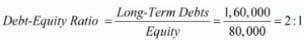Page No. 4.95

Question:35
From the following information, calculate Debt to Equity Ratio: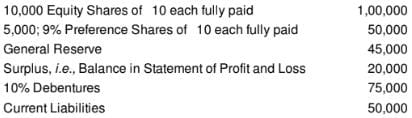Solution:
Long-Term Debt = Debentures = Rs 75,000
Shareholder’s Funds = Equity Share Capital + Preference Share Capital + General Reserve + Surplus
= Rs 1,00,000 + Rs 50,000 + Rs 45,000 + Rs 20,000 = Rs 2,15,000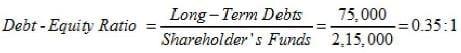Question:36
When Debt to Equity Ratio is 2, state giving reason, whether this ratio will increase or decrease or will have no change in each of the following cases:

(i) Sale of Land Bookvalue 4, 00, 000 for 5,00,000;
(ii) Issue of Equity Shares for the purchase of Plant and Machinery worth
10,00,000;
(iii) Issue of Preference Shares for redemption of 13% Debentures, worth 10,00,000.
Solution:

Debt-Equity Ratio = 2:1
Let Long-term loan = Rs 20,00,000
Shareholders’ Funds = Rs 10,00,000
(i) Sale of Land BookValueRs4, 00, 000 for Rs 5,00,000- Decrease
Reason: This transaction will result increase in Shareholders’ Funds by Rs 1,00,000 as profit on sale of Land.
Shareholders’ Funds after adjusting profit on sale of land = 10,00,000 + 1,00,000 = Rs 11,00,000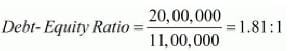(ii) Issue of Equity share for the purchase of plant and Machinery worth Rs 10,00,000- Decrease
Reason: This transaction will increase the amount of Shareholders Fund by Rs 10,00,000 in the form of equity shares and have no effect on Long-term Loans.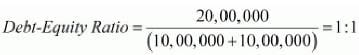(iii) Issue of preference Shares for redemption of 13% Debentures worth Rs 10,00,000- Decrease
Reason: This transaction will lead to decrease in Long-term Loan by Rs 10,00,000 in the form of redemption of debentures and increase in Shareholders’ Funds with the same amount in the form of Preference Shares.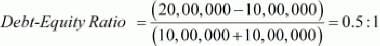Question:37
Total Assets 12,50,000; Total Debts 10,00,000; Current Liabilities
5,00,000. Calculate Debt to Equity Ratio.
Solution:
Total Assets = 12,50,000
Total Debts = 10,00,000
Equity = Total Assets − Total Liabilities
= 12,50,000 − 10,00,000 = 2,50,000
Long-term Debts = Total Debts − Current Liabilities
= 10,00,000 − 5,00,000 = 5,00,000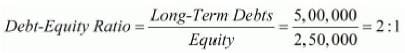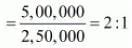Question:38
Capital Employed 8,00,000; Shareholders' Funds 2,00,000. Calculate Debt to Equity Ratio.
Solution:

Shareholders’ Funds = 2,00,000
Capital Employed = 8,00,000
Long- Term Debts = Capital Employed − Shareholders’ Funds
= 8,00,000 − 2,00,000 = 6,00,000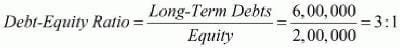Question:39
Balance Sheet had the following amounts as at 31st March, 2019: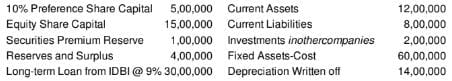Calculate ratios indicating the Long-term and the Short-term financial position of the company.

Solution:
(i) Debt-Equity Ratio is an indicator of Long-term financial health. It shows the proportion of Long-term loan in comparison of shareholders’ Funds.
Debt - Equity Ratio =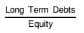Debt = Loan from IDBI @ 9% = 30,00,000
Equity = 10% Preference Share Capital + Equity Share Capital + Reserves & Surplus
= 5,00,000 + 15,00,000 + 4,00,000 = 24,00,000
Debt - Equity Ratio =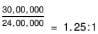(ii) Current Ratio is an indicator of short-term financial portion. It shows the proportion of Current Assets in comparison of Current Liabilities.
Current Ratio =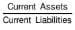Current Assets = 12,00,000
Current Liabilities = 8,00,000
Current Ratio =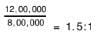Note: In the above question, Securities Premium Reserve is not considered while computing Equity because it is already included in the amount of Reserves and Surplus.

Question:40 Calculate Debt to Equity Ratio from the following information:
Fixed Assets Gross           8,40,000        Current Assets 3,50,000
Accumulated Depreciation 1,40,000        Current Liabilities 2,80,000
Non-current Investments    14,000          10% Long-term Borrowings 4,20,000
Long-term Loans and Advances 56,000    Long-term Provisions 1,40,000

Solution:
Debt = Long Term Borrowings + Long Term Provisions
= 4,20,000+1,40,000 = Rs 5,60,000
Equity = Total Assets - Total Debts
= (8,40,000 -1,40,000+14,000+56,000+3,50,000) - (4,20,000 -1,40,000
-2,80,000)= Rs 2,80,000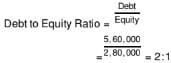Question:41
Debt to Equity Ratio of a company is 0.5:1. Which of the following suggestions would increase, decrease or not change it:
(i) Issue of Equity Shares:
(iii) Redemption of debentures;
(iv)  Purchased goods on Credit?
Solution:

Debt Equity Ratio = 0.5:1
Let Long- term Loan be = Rs 5,00,000
Shareholders’ Funds = Rs 10,00,000
(i) Issue of Equity shares- Decrease
Reason: Issue of equity shares results in increase in Shareholders’ Funds in the form of equity shares but there will be no change in Long-term Loan.
Example: Issue of equity share Rs 5,00,000
Shareholders’ Funds after issue of equity shares = 10,00,000 + 15,00,000
= Rs 15,00,000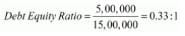(ii) Cash received from Debtors- No Change
Reason: Cash received from debtors will increase one current asset in the form of cash and decrease other asset in the form of debtors. This transaction will have no effect on Long-term Loan and Shareholders’ Funds.
(iii) Redemption of Debentures- Decrease
Reason: This transaction will result decrease in Long-term Loans in the form of reduction in debtors and no change in Shareholders’ Funds.
Example: Redemption of Debentures Rs 2,00,000
Long-term Loan = 5,00,000 − 2,00,000 = 3,00,000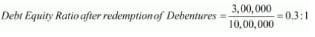(iv) Purchased of goods on Credit- No Change
Reason: Neither Long-term loan nor share holders’ funds will be affected by this transaction because purchase of goods results no change in Long-term Loan and Shareholders’ Funds.

Question:42
Assuming That the Debt to Equity Ratio is 2 : 1, state giving reasons, which of the following transactions would
(i) increase;
(ii) Decrease;
(iii) Not alter Debt to Equity Ratio:

(i) Issue of new shares for cash.
(ii) Conversion of debentures into equity shares
(iii) Sale of a fixed asset at profit.
(iv) Purchase of a fixed asset on long-term deferred payment basis.
(v) Payment to creditors.
Solution:
Let’s take Debt and Equity as Rs 2,00,000 and Rs 1,00,000
Debt to Equity Ratio = Debt/Equity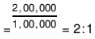(i) Issue of new shares for cash say Rs 50, 000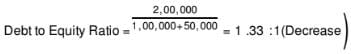(ii) Conversion of debentures into equity shares say Rs 50, 000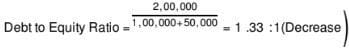(iii) Sale of a fixed asset at profit say R s50, 000 profit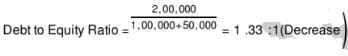(iv) Purchase of fixed asset on long term payment basis say Rs50, 000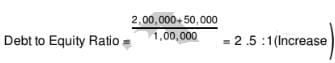(v)  Payment to creditors say Rs50, 000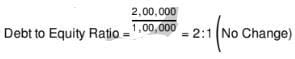Question:43
From the following Balance Sheet of ABC Ltd. as at 31st March, 2019, Calculate Debt to Equity Ratio: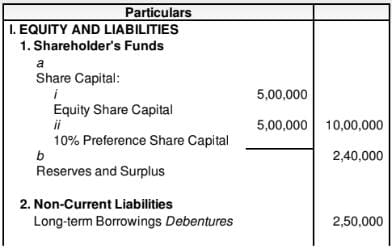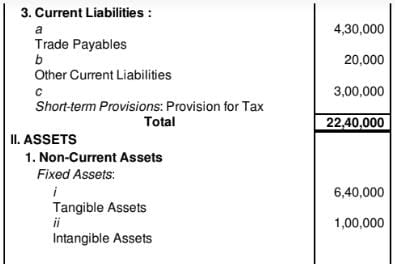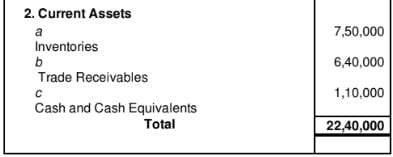Solution:
Long-term Debt = Debentures = 2,50,000
Equity = Equity Share Capital + 10% Preference Share Capital + Reserves and Surplus
= 5,00,000 + 5,00,000 + 2,40,000 = 12,40,000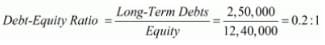Question:44
Calculate Total Assets to Debt Ratio from the following information:
Long-term Debts 4,00,000; total Assets 7,70,000.
Solution:
Long-term Debts = 4,00,000
Total Assets = 7,70,000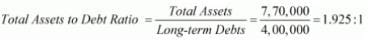Question:45 Shareholders' Funds 1,60,000; Total Debts 3,60,000; Current Liabilities 40,000. Calculate Total Assets to Debt Ratio.
Solution:
Total Debts = 3,60,000
Shareholders’ Funds = 1,60,000
Current Liabilities = 40,000
Total Assets = Total Debts + Shareholders’ Funds
= 3,60,000 + 1,60,000 = 5,20,000
Long-term Debts = Total Debt − Current Liabilities
= 3,60,000 − 40,000 = 3,20,000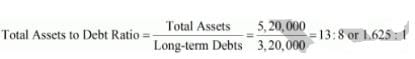Question:46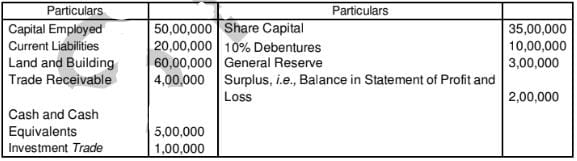Solution:
Total Assets to Debt Ratio = Total Assets/Long Term Debt
Total Assets = Land and Buildings + Trade Receivables + Cash and Cash Equivalents + Investments Trade
= 60,00,000 + 4,00,000 + 5,00,000 + 1,00,000
= Rs 70, 00,000
Long Term Debts = Capital Employed - Shareholders’ funds
= 50,00,000 – 40,00,000
= Rs 10,00,000
Shareholder’s Fund = Share Capital + Reserve and Surplus
= 35,00,000 + 3,00,000 + 2, 00,000
= Rs 40,00,000
Total Assets to Debt Ratio =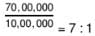Question:47 Total Debt 60,00,000; Shareholders' Funds 10,00,000; Reserves and Surplus 2,50,000; Current Assets 25,00,000; Working Capital 5,00,000. Calculate Total Assets to Debt Ratio.
Solution:

Total Assets to Debt Ratio =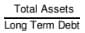Working Capital = Current Assets – Current Liabilities
5,00,000 = 25,00,000 – Current Liabilities
Current Liabilities = Rs 20,00,000
Long Term Debts = Total Debt – Current Liabilities
= 60,00,000 – 20,00,000
= Rs 40,00,000
Total Assets = Total Liabilities = Total Debt + Shareholders’ Funds
= 60,00,000 + 10,00,000
= Rs 70,00,000
Total Assets to Debt Ratio =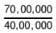= 7 : 4 or 1.75 : 1

Question:48 Total Debt 15,00,000; Current Liablities 5,00,000; Capital Employed 15,00,000. Calculate Total Assets to Debt Ratio.
Solution:

Total Assets to Debt Ratio =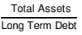Capital Employed = Total Assets – Current Liabilities
15,00,000 = Total Assets – 5,00,000
Total Assets = Rs 20,00,000
Long Term Debt = Total Debt – Current Liabilities
= 15,00,000 – 5,00,000
= Rs 10,00,000
Total Assets to Debt Ratio =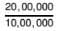= 2 : 1

Question:49 Calculate Total Assets to Debt Ratio from the following information: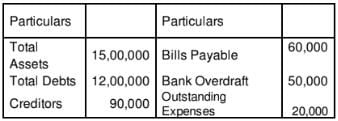Solution:

Total Assets = Rs 15,00,000
Current Liabilities = Creditors + Bills Payable + Bank Overdraft + Outstanding Expenses
= Rs 90,000 + Rs 60,000 + Rs 50,000 + Rs 20,000 = Rs 2,20,000
Long-Term Debt = Total Debt – Current Liabilities
= Rs 12,00,000 – Rs 2,20,000 = Rs 9,80,000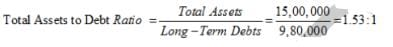Question:50 Total Debt 12,00,000; Shareholders' Funds 2,00,000; Reserves and Surplus 50,000; Current Assets 5,00,000; Working Capital 1,00,000. Calculate Total Assets to Debt Ratio.
Solution:

Working Capital = Current Assets - Current Liabilities
1,00,000 = 5,00,000 - Current Liabilities
Current Liabilities = Rs 4,00,000
Debt = Total Debt - Current Liabilities = 12,00,000 - 4,00,000 = Rs 8,00,000
Total Assets = Shareholders' Funds+ Total Debt
= 2,00,000 + 12,00,000 = Rs 14,00,000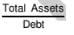Total Assets to Debt Ratio =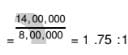The document Accounting Ratios (Part - 2) Notes | Study TS Grewal Solutions - Class 12 Accountancy - Commerce is a part of the Commerce Course TS Grewal Solutions - Class 12 Accountancy.
All you need of Commerce at this link: CommerceUse Code STAYHOME200 and get INR 200 additional OFF

## TS Grewal Solutions - Class 12 Accountancy

52 docs

Track your progress, build streaks, highlight & save important lessons and more!

,

,

,

,

,

,

,

,

,

,

,

,

,

,

,

,

,

,

,

,

,

;